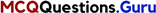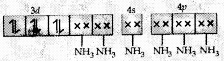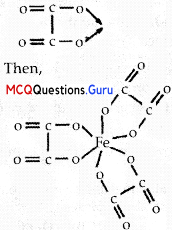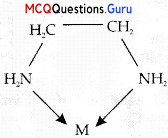# MCQ Questions for Class 12 Chemistry Chapter 9 Coordination Compounds

## Coordination Compounds Class 12 MCQs Questions with Answers

Coordination Compounds Class 12 MCQ Chapter 9 Question 1.

## The oxidation of Ni in [Ni(CO)4] is ………….

(A) 0
(B) 2
(C) 3
(D) 4
(A) 0

Explanation:
CO is a neutral ligand and ils oxidation state is zero Since the overall charge on the complex is zero too, hence oxidation State of Ni is 0.

Coordination Compounds MCQ Chapter 9 Question 2.

## Which of the following will give a white precipitate upon reacting with AgNO3?

(A) K2[Pt(en)2Cl2]
(B) [Co(NH3)3Cl3]
(C) [Cr(H2O)6]Cl3
(D) [Fe(H2O)3Cl3]

(C) [Cr(H2O)6]Cl3

Explanation:
AgNO3+ Cr(H2O)6]Cl3 → AgCl ppt should he below AgCl + NO3, Since Cl is outside the coordination sphere, it 1 scan read with AgNOj forming the while AgCl precipitate.

MCQ Of Coordination Compounds Class 12 Chapter 9 Question 3.

## The formula of the complex triamminetri(nitrito – O) Cobalt (III) is

(A) [CO(ONO)3(NH3)3]
(B) [CO(NO2)3(NH3)3]
(C) [CO(ONO2)3(NH3)3]
(D) [CO(NO2)(NH3)3]
(A) [CO(ONO)3(NH3)3]

Explanation:
[CO(ONO)3(NH3)3]MCQ On Coordination Compounds Chapter 9 Question 4.

## How many ions are produced from the complex [Co(NH3)5Cl]Cl2in solution?

(A) 4
(B) 2
(C) 3
(D) 5
(C) 3

Explanation:
[Co(NH3)5Cl]Cl2 → [CO(NH3)5Cl](aq) + 2Cl

Coordination Compounds MCQ With Answers Pdf Chapter 9 Question 5.

## The pair [CO(NH3)4Cl2]Br2 and [CO(NH3)4Br2]Cl2 will show

(B) Hydrate isomerism
(C) Ionization isomerism
(D) Coordinate isomerism
(C) Ionization isomerism

Explanation:
loni/ation isomer’, have identical central ion and the other ligands except lor a ligand that has exchanged places with an anion or neutral molecule lhat was originally outside the coordination complex.
[Co(NH3)4Cl]Br2 → [Co(NH3)4Cl2]+ + 2Br
[Co(NH3)4Br2]Cl2 →[Co(NH3)4Cl2]+ + 2Cl

Coordination Chemistry MCQ With Answers Chapter 9 Question 6.

## The coordination number of ‘Co’ in the complex [Co(en)3]3+ is

(A) 3
(B) 6
(C) 4
(D) 5
(B) 6

Explanation:
Coordination number is the number of ligands joined to the central metal ion or atom. Since ethvlenediamine is a bidentate ligand, Co has coordination number of 6.Coordination Compounds MCQ With Answers Chapter 9 Question 7.

## Which of the following is the most stable complex?

(A) [Fe(CO)5]
(B) [Fe(H20)6]3+
(C) [Fe(C204)3]3
(D) [Fe(CN)6]3
(C) [Fe(C204)3]3

Explanation:
[Fe(C204)3]3+ acts as the chelating ligands, because C2O4is a bidentate ligand.

MCQ On Coordination Compounds Class 12 Chapter 9 Question 8.

## What type of isomerism is shown by the pair [Cr(H2O)6]Cl3 and [Cr(H2O)5Cl] Cl2. H2O ?

(A) Ionisation isomerism
(B) Coordination isomerism
(C) Solvate isomerism
(C) Solvate isomerism

Explanation:
Solvate / Hydrate isomerism Solvate or hvdrate isomers have the same ^composition but differ with respect to the number of solvent ligand molecules as well as the counter ion in the crystal lattice.

Coordination Compounds MCQs Chapter 9 Question 9.

## Ambidentate ligands like NO–2 and SCN– are:

(a) unidentate
(b) didentate
(c) polydentate
(d) has variable denticity

OR

## The formula of the coordination compound Tetraam mineaquachloridocobalt(III) chloride is

(A) [Co(NH3)4(H20)Cl]Cl2
(B) [Co(NH3)4(H20)Cl]Cl3
(C) [CO(NH3)2(H20)C1]Cl2
(D) [Co(NH3)4(H20)Cl]Cl
(A) [Co(NH3)4(H20)Cl]Cl2

Explanation:
Tetraammineaquachloridocobalt (III) chloride is [C0(NH3)4(H2O)Cl]Cl2.
Central atom – Cobalt (III) Coordination sphere ligands – Tetraammince – 4 NH3 groups neutral ligand Aqua – 1 H2O groups neutral ligand Chlorido -1 Cl group, negatively charged ligand, one negative charge Counter ion- Chloride ions, 2 Since Cobalt is 3+, one valency is satisfied with , Cl in coordination sphere and 2 bv chlorine n counter ions. Hence, the formula of the coordination compound is [CO(NH3)4(H2O)Cl]Cl2.Class 12 Chemistry Chapter 9 MCQ Question 10.

## The crystal field splitting energy for octahedral (∆0) and tetrahedral (∆t) complexes is related as

(A) $$\Delta_{t}=\frac{2}{9} \Delta_{0}$$
(B) $$\Delta_{t}=\frac{5}{9} \Delta_{o}$$
(C) $$\Delta t=\frac{4}{9} \Delta_{o}$$
(D) $$\Delta_{t}=2 \Delta_{o}$$
(C) $$\Delta t=\frac{4}{9} \Delta_{o}$$

Explanation:
$$\Delta t=\frac{4}{9} \Delta_{o}$$

Coordination Compound MCQ Chapter 9 Question 11.

## Atomic number of Mn, Fe and Co are 25, 26, 27 respectively. Which of the following inner orbital octahedral complex ions are diamagnetic?

(A) [Co(NH3)6]3+
(B) [Mn(CN)6]3-
(C) [Fe(CN)6]3-
(D) None of the above
(A) [Co(NH3)6]3+

Explanation:
Molecular orbital electronic configuration of Co3+ in [Co(NH3)6]3+ isNumber of unpaired electron = 0
Magnetic property = Diamagnetic

MCQs On Coordination Compounds Chapter 9 Question 12.

## The colour of the coordination compounds depends on the crystal field splitting. What will be the correct order of absorption of wavelength of light in the visible region, for the complexes, [CO(NH3)6]3+, [CO(CN)6]3-, [CO(H20)6]3+

(A) [CO(CN)6]3- > [CO(NH3)6]3+ > [CO(H20)6]3+
(B) [CO(NH3)6]3+> [CO(H20)6]3+ > [CO(CN)6]3-
(C) [CO(H2O)6]3+ > [CO(NH3)6]3+ > [CO(CN)6]3+
(D) [CO(CN)6]3+ > [Co(NH3)6]3+ > [CO(H20)6]3+
(C) [CO(H2O)6]3+ > [CO(NH3)6]3+ > [CO(CN)6]3+

Explanation:
0 values follow the order: [CO(H2O)6]3+ < [Co(NH3)6]3+ < [Co(CN)6]3- and therefore, absorption wavelength follows the order: [Co(NH3)6]3+ >[Co(NH3)6]3+ >[Co(CN)6]3-Coordination Chemistry MCQ Chapter 9 Question 13.

## Which of the following complexes formed by Cu ions is most stable?

(A) Cu2+ + 4NH3 → [Cu(NH3)4]2+, log K = 11.6
(B) Cu2+ + 4CN → [Cu(CN4)]2-, logK = 27.3
(C) Cu2+ + 2en → [Cu(en)2]2+, log K = 15.4
(D) Cu2+ + 4H60 → [Cu(H2O)4]2+, log K = 8.9
(D) Cu2+ + 4H60 → [Cu(H2O)4]2+, log K = 8.9

Explanation:
The greater the value of log K, the greater will be stability of complex compound formed. For reaction, log K has the highest value.
Cu2+ + 4CN0 → [CU(CN)4]2+ ;
K = $$\frac{\left[\mathrm{Cu}(\mathrm{CN})_{4}\right]^{2+}}{\left[\mathrm{Cu}^{2+}\right]\left[\mathrm{CN}^{-}\right]^{4}}$$ and log K = 27.3

MCQ On Coordination Chemistry Chapter 9 Question 14.

## The CFSE for octahedral [CoCl6]4- is 18,000 cm-1 The CFSE for tetrahedral (CoCl4]2- will be

(A) 18,000 cm-1
(b) 16,000 cm-1
(C) 8,000 cm-1
(d) 20,000 cm-1
(C) 8,000 cm-1

Explanation:
CFSE for tetrahedral complex is
$$\Delta_{1}=\frac{4}{9} \Delta_{0}$$
$$\frac{4}{9} \times 18,000$$ = 8,000 cm-1

MCQ Questions On Coordination Compounds Chapter 9 Question 15.

## Amongst the following, the most stable complex is

(A) [Fe(H4O)6]3
(B) [Fe(NH3)6]3+
(C) [Fe(C2O4)33-
(D) [FeCl6]3-
(C) [Fe(C2O4)33-

Explanation:
We know that the stability of a complex increases by chelation. Therefore, the most stable complex is [Fe(C2O4)33-.Class 12 Coordination Compounds MCQ Chapter 9 Question 16.

## How many ions are produced from the complex CO(NH3)6Cl2 in solution?

(A) 6
(B) 4
(C) 3
(D) 2
(D) 2

Explanation:
The given complex can be written as CO(NH3)6Cl2
Thus,
[CO(NH3)6]+ along o Cl ions are produced.Assertion And Reason Based MCQs

Directions: In the following questions, A statement of Assertion (A) is followed by a statement of Reason (R). Mark the correct choice as.
(A) Both A and R are true and R is the correct explanation of A
(B) Both A and R are true but R is NOT the correct explanation of A
(C) A is true but R is false
(D) A is false and R is true

Coordination Compounds MCQ Pdf Chapter 9 Question 1.

## Assertion (A): Toxic metal ions are removed by the chelating ligands. Reason (R): Chelate complexes tend to be more stable.

(A) Both A and R are true and R is the correct explanation of A

Explanation:
When a solution of chelating ligand is added lo solution containing toxic metal ligands chelates the metal ions bv formation of I, stable complex.

Chapter 9 Chemistry Class 12 MCQs Question 2.

## Assertion (A): Linkage isomerism arises in coordi-nation compounds containing ambidentate ligand. Reason (R): Ambidentate ligand has two different donor atoms.

(A) Both A and R are true and R is the correct explanation of A

Explanation:
Linkage isomerism arises due to two different donor atoms in ambidentate ligand.Question 3.

## Assertion: Among [CO(NH3)6]3+ and [CO(en)3]3+, coordination compound [CO(en)3]3+ is a more stable complex. Reason: Because (en) is a chelating ligand/bidentate ligand.

(A) Both A and R are true and R is the correct explanation of A

Explanation:
Since (ethylene diamine-en) is a chelating ligand/bidentate ligand, [CO(en3)]3+ is a more stable complex as compared to the other one.Question 4.

## Assertion (A): [Fe(CN)6]3- ion shows magnetic moment corresponding to two unpaired electrons. Reason (R): Because it has d2sp3 type hybridisation.

(D) A is false and R is true

Explanation:
[Fe(CN)6]3- ion shows magnetic moment corresponding to two unpaired electrons.

Question 5.

## Assertion (A): [Cr(H20)6]Cl2 and [Fe(H20)6]Cl2 are reducing in nature. Reason (R): Unpaired electrons are present in their d-orbitals.

(B) Both A and R are true but R is NOT the correct explanation of A

Explanation:
In the complexes, Co exists as Co2+ and Fe as Fe2+. Both of the complexes become Stable by oxidation of metal ion to Co3+ and Fe3+

Question 6.

## Assertion (A): Complexes of MX6 and MX5L type (X and L are unidentate) do not show geometrical isomerism. Reason (R): Geometrical isomerism is not shown by complexes of coordination number 6.

(B) Both A and R are true but R is NOT the correct explanation of A

Explanation:
For complexes of MX6 and MX5L Type, different geometric arrangements of the ligands are not possible due to presence of plane of symmetry.Question 7.

## Assertion (A): Low spin tetrahedral complexes are rarely observed. Reason (R): Crystal field splitting is less than pairing energy for tetrahedral complexes.

(A) Both A and R are true and R is the correct explanation of A

Explanation:
In tetrahedral complexes, the splitting of the d-orbitals is inverted and is smaller in comparison to octahedral complexes. The Crystal field splitting energy is not large enough to force pairing and hence, low spin complexes are rarely observed.

Case-Based MCQs

The crystal field theory (CFT) is an electrostatic model which considers the metal-ligand bond to be ionic arising purely from electrostatic interactions between the metal ion and the ligand. Ligands are treated as point charges in case of anions or dipoles in case of neutral molecules.

The five d orbitals in an isolated gaseous metal atom ion have same energy, i.e., they are degenerate. This degeneracy is maintained if a spherically symmetrical field of negative charges surrounds the metal atom/ ion. However, when this negative field is due to ligands (either anions or the negative ends of dipolar molecules like NH3 and H20) in a complex, it becomes asymmetrical and the degeneracy of the d orbitals is lifted. It results in splitting of the d orbitals.

The following questions are multiple choice questions. Choose the most appropriate answer:

Question 1.

## The colour of the coordination compounds depends on the crystal field splitting. What will be the correct order of absorption of wavelength of light in the visible region, for the complexes, [CO(NH3)6]3+, [CO(CN)6]3-, [CO(H20)6]3+

(A) [CO(CN)6]3- > [CO(NH3)6]3+ > [CO(H2O)6]3+
(B) [CO(NH3)6]3+ > [CO(H2O)6]3+ > [CO(CN)6]3-
(C) [CO(H2O)6]3+ > [CO(NH3)6]3+ > [CO(CN)6]3-
(D) [CO(CN)6]3-> [CO(NH3)6]3+> [CO(H2O)6]3+
(C) [CO(H2O)6]3+ > [CO(NH3)6]3+ > [CO(CN)6]3-

Explanation:
(C) [CO(H2O)6]3+ > [CO(NH3)6]3+ > [CO(CN)6]3-

Question 2.

## The CFSE for octahedral [CoCl6]4- is 18,000 cm-1. The CFSE for tetrahedral [CoCl4]2- will be

(A) 18,000 cm-1
(B) 16,000 cm-1
(C) 8,000 cm-1
(D) 20,000 cm-1
(C) 8,000 cm-1

Explanation:
8,000 cm-1Question 3.

## An aqueous pink solution of cobalt(II) chloride changes to deep blue on addition of excess of HCl. This is because .

(A) [Co(H20)6]2+ is transformed into [CoCl6]4
(B) [Co(H20)6]2+ is transformed into [CoCl4]2-
(C) tetrahedral complexes have larger crystal field splitting than octahedral complex.
(D) None of the above
(B) [Co(H20)6]2+ is transformed into [CoCl4]2-

Explanation:
[Co(H2O)6]2+ is transformed into [CoCl4]2-.

Question 4.

## A chelating agent has two or more than two donor atoms to bind to a single metal ion. Which of the following is not a chelating agent?

(A) thiosulphato
(B) oxalato
(C) glycinato
(D) ethane-1,2-diamine
(A) thiosulphato

Explanation:
Thiosulphato is a mnnodentate ligand whereas Oxalato, glycinaLo and elhvlene diamine are bidentate ligands and can form rings with the central metal ion. So, they are also chelating ligands. Thiosulphato is a monodentale ligand and hence, cannot form chelate rings. Hence, it is not a chelating ligand

According to Valence Bond Theory, the metal atom or ion under the influence of ligands can use its (n – l)d, ns, np or ns, np, nd orbitals for hybridisation to yield a set of equivalent orbitals of definite geometry such as octahedral, tetrahedral, square planar and so on. These hybridised orbitals are allowed to overlap with ligand orbitals that can donate electron pairs for bonding.

In these questions a statement of assertion followed by a statement of reason is given. Choose the correct answer out of the following choices.
(A) Assertion and reason both are correct statements and reason is correct explanation for assertion.
(B) Assertion and reason both are correct statements but reason is not correct explanation for assertion.
(C) Assertion is correct statement but reason is wrong statement
(D) Assertion is wrong statement but reason is correct statement.

Question 1.

## Assertion (A): In the diamagnetic octahedral complex, [CO(NH3)6]2+, the cobalt ion is in +3 oxidation state. Reason (R): Six pairs of electrons, one from each NH3 molecule, occupy the six hybrid orbitals.

(B) Assertion and reason both are correct statements but reason is not correct explanation for assertion.

Explanation:
In the diamagnetic octahedral complex, [Co(NH3)6]3+, the cobalt ion is in +3 oxidation state and has the electronic configuration 36.Question 2.

## Assertion (A): [NiCl4]2-is an inner orbital complex. Reason (R): An inner orbital or low spin or spin paired complex uses inner d orbitals of the metal ion for hybridisation.

(D) Assertion is wrong statement but reason is correct statement.

Explanation:
[NiCl4]2- is high spin complex.

Question 3.

## Assertion (A): In the square planar complexes, the hybridisation involved is dsp . Reason (R): In [Ni(CN)4]2- Here nickel is in +2 oxidation state and has the electronic configuration 3d8.

(B) Assertion and reason both are correct statements but reason is not correct explanation for assertion.

Question 4.

## Assertion (A): The paramagnetic octahedral complex, [COF6]3- uses outer orbital (4d) in hybridisation (sp3d2). Reason (R): It is a high spin complex.

(C) Assertion is correct statement but reason is wrong statement

Explanation:
The paramagnetic octahedral complex, [COF6]3- uses outer orbital (4d) in hybridisation (sp3d2). It is a low spin complex.

The existence of coordination compounds with the same formula but different arrangements of the ligands was crucial in the development of coordination chemistry. Two or more compounds with the same formula but different arrangements of the atoms are called isomers. Isomers are compounds with the same molecular formula but different structural formulas and do not necessarily share similar properties.

There are many different classes of isomers, like stereoisomers, enantiomers, and geometrical isomers. There are two main forms of isomerism: structural isomerism and stereoisomerism. The different chemical formulas in structural isomers are caused either by a difference in what ligands are bonded to the central atoms or how the individual ligands are bonded to the central atoms.The following questions are multiple choice questions. Choose the most appropriate answer:

Question 1.

## What kind of isomerism exists between [Cr(H40)6] Cl3 (violet) and [Cr(H40)5Cl]Cl2.H20 (greyish- green)?

(B) solvate isomerism
(C) ionisation isomerism
(D) coordination isomerism
(B) solvate isomerism

Question 2.

## Which of the following complexes show linkage isomerism?

(A) [CO(H20)5CO]3+
(B) [Cr(NH3)5SCN]2+
(C) [Fe(en)2Cl2]+
(D) All of the above
(B) [Cr(NH3)5SCN]2+

Explanation:
Linkage isomerium is the existence of coordination compounds that have the same composition differing with the connectivity of the metal to a ligand. Typical ligands that give rise to linkage isomers are: thiocyanate, SCN – isothiocvanate, .NCS –

Question 3.